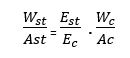# Analysis of Composite Sections by Elastic Theory ( Working Stress Method)

Analysis of composite sections by elastic theory literally means the design based on working stress method(WSM). This article explains the the starting of design analysis of concrete structures as per WSM method. By the end of this article, you will study:
1. To find steel member takes more load than Concrete in R.C.C.
2. Equivalent or Transformed Concrete Area
3. Insights on Composite Member Subjected to Bending
If you need proper lead on this article, you can visit the article: What is Working Stress Method? Assumptions, Features, Merits & Demerits.

This analysis is performed to study how much load is taken by steel and concrete in a R.C structure when subjected to external loading. The analysis also gives a relation to determine, the equivalent concrete area to be used if steel is minimized.
Consider a reinforced concrete section (R.C.C) as shown in the figure-1 below. For convenient analysis and better understanding, the steel and concrete has been illustrated separately. The given measurements are:

1. Area of Steel Asc
2. Area of Concrete Ac
3. The load Applied on the Whole Unit W
4. The load taken by Steel Ws
5. The load taken by Concrete WcFig.1. Steel and Concrete  ( RC Structure) Illustrated separately under the Action of Load W
Under the action of loads, both the steel and concrete section is subjected to strain in equal amounts. They have strain compatibility as per the Assumptions of Working Stress Method.
Then,
Strain in Steel = Strain in ConcreteEq.1
In the above relation, est  and e are the strain in steel and concrete. Stress in steel and concrete is given by σst and σc respectively. Then we have relationship between stress and strain as: Strain (e) = Stress/Modulus of Elasticity (E). Where, Stress is again given by load (W) divided by Area of cross-section (A). Est and Ec are the modulus of elasticity of Steel and concrete.

We know that,
Modular Ratio (m) = Es/Ec

From the Equation-1, it can be given that,Relation-2

From the above relation, we can conclude:

Es/Em = m= modular ration between steel and Concrete

## How to Prove Steel Member is Subjected to Greater load than Concrete?

From the Equation 1, it is clear that Wst + Wc = W, Where 'W' is the total Load. Hence the following relation can be obtained:

Eq.4

Also, Est = mEc, by substituting in Eq.4, we get:

Suppose the areas Ast = Ac; Then we get the relation,

For a modular ration value m=18, the areas Ast = Ac; When substituting in eq.6, we get:
Wc = W/19;
This proves that the steel member us subjected to a greater load than the concrete.

## What is Equivalent or Transformed Concrete Area of Steel reinforcement?

We know that:
W = Wst + Wc
W = σstAst + σc Ac   Eq.7

But we know that:
σst= (Es/Ec)σc = mσc
Substituting the above relation in Eq.7, we get
W= mσc Ast + σc Ac;
Hence,
Here,
Equivalent Concrete Area = Ae = Actual Concrete Area + m x steel area = mAst + Ac ( Denominator of the Above Equation)

If you wish to watch the Video Tutorial Please click the Link Below

FOR MORE CIVIL ENGINEERING NOTES AND EXPLANATIONS KINDLY